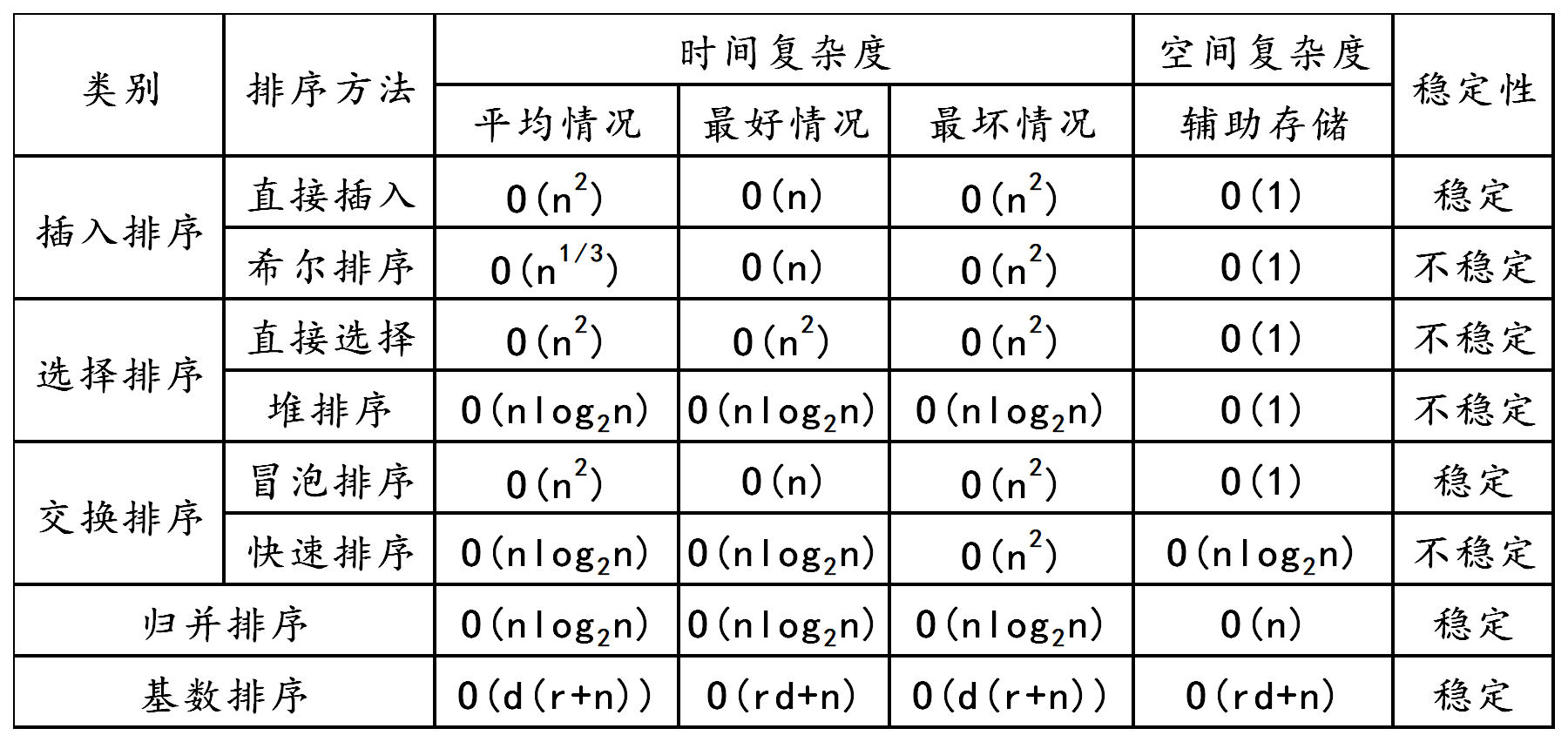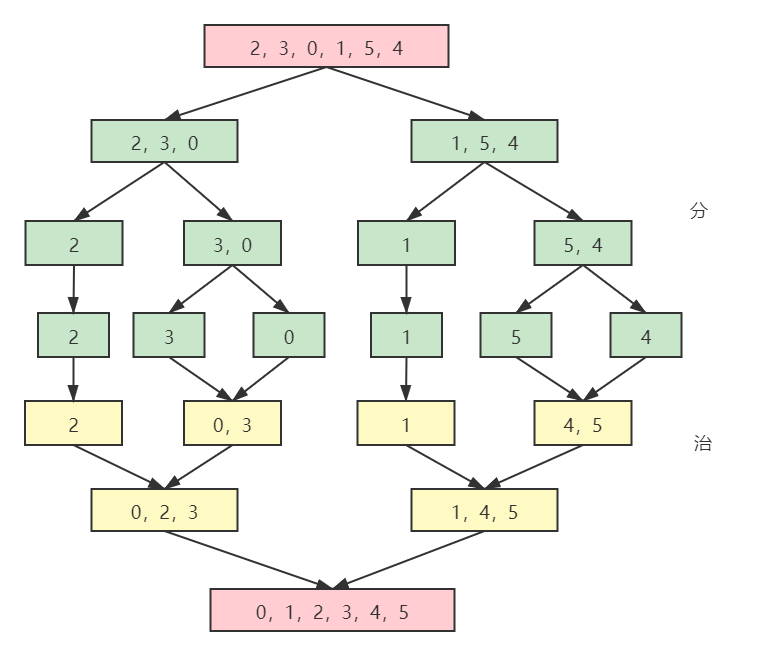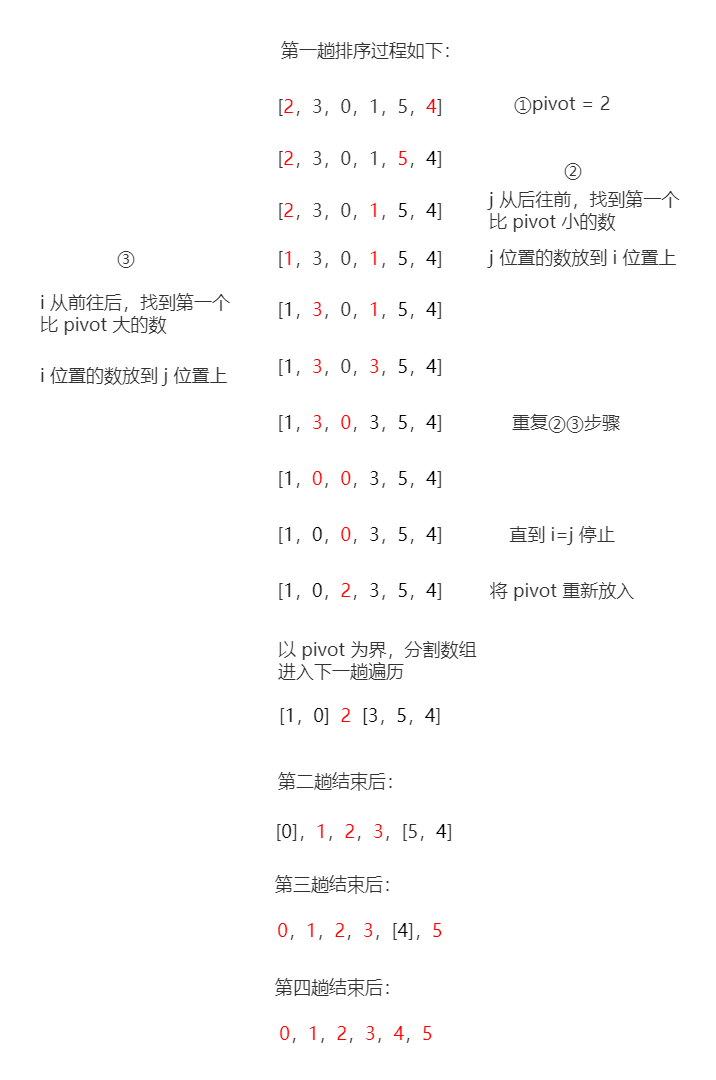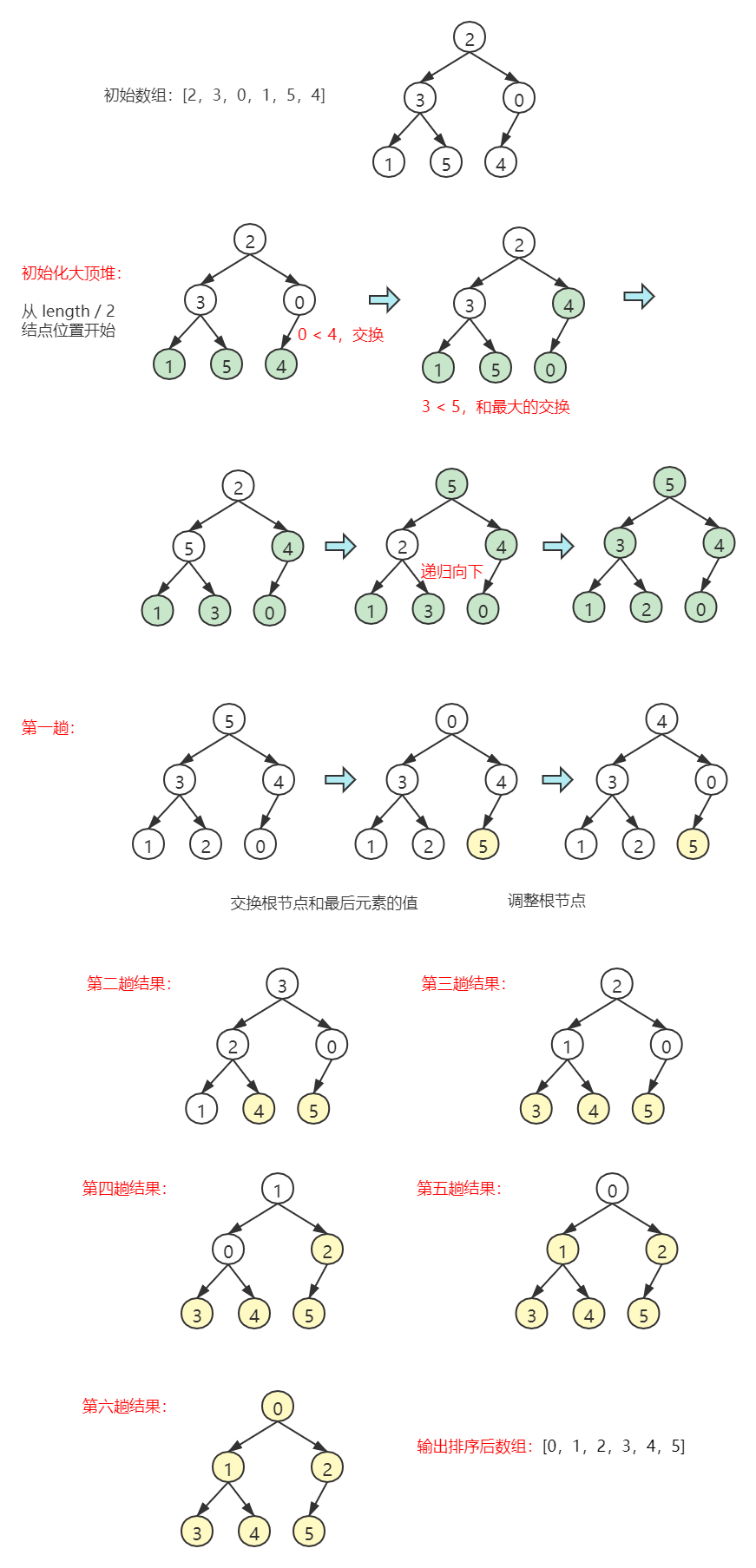• 各内排序算法时空复杂度对比表 ...

目录
各内排序算法时空复杂度对比表
源码

各内排序算法时空复杂度对比表
算法最好平均最好空间复杂稳定插入O(n)O(n²)←O(1)是选择O(n²)←←↑ 冒泡O(n)O(n²)←↑是希尔-O(n1.3)-↑ 快排O(nlog₂n)←O(n²)O(log₂n) 堆排↑←←O(1) 归并↑←←O(n)是基数O(d(n+r))←←O(r)是
源码
package top.senseiliu.codesnippet;

public final class SortUtils {
private SortUtils() {

}

// 输出数组
public static void printNum(int[] num) {
for (int item : num) {
System.out.print(item + " ");
}
System.out.println();
}

/**
* 直接插入法
* 从第二个元素开始遍历(正序)
* 对当前元素向前遍历(倒序)
*   如果前一个值大于当前值，把当前位置值置为前一个值，继续向前遍历
* 最后把当前值插回去
*
* @param num
*/
public static void insertSort(int[] num) {
for (int i = 1; i < num.length; ++i) {
int curNum = num[i];
int preIndex = i - 1;
while (preIndex >= 0 && num[preIndex] > curNum) {
num[preIndex + 1] = num[preIndex];
--preIndex;
}
num[preIndex + 1] = curNum;
}
}

/**
* 简单选择法
* 从第一个元素遍历到倒数第二个
* 从当前元素下标的下一个下标开始遍历，得到一个最小值的下标
* 如果当前元素下标不等于当前元素下标，则交换两值
*
* @param num
*/
public static void selectSort(int[] num) {
for (int i = 0; i < num.length - 1; ++i) {
int minIndex = i;
for (int j = i + 1; j < num.length; ++j) {
if (num[j] < num[minIndex]) {
minIndex = j;
}
}
if (i != minIndex) {
int minValue = num[minIndex];
num[minIndex] = num[i];
num[i] = minValue;
}
}
}

/**
* 冒泡排序法-普通版
*
* @param num
*/
public static void bubbleSort(int[] num) {
for (int i = 0; i < num.length - 1; ++i) {
for (int j = num.length - 1; j > i; --j) {
if (num[j - 1] > num[j]) {
int tmp = num[j];
num[j] = num[j - 1];
num[j - 1] = tmp;
}
}
}
}

/**
* 冒泡排序法-优化版
* 一次都没有产生交换说明数组基本有序直接退出
*
* @param num
*/
public static void bubbleSortOptimized(int[] num) {
boolean sorted;
for (int i = 0; i < num.length - 1; ++i) {
sorted = true;
for (int j = num.length - 1; j > i; --j) {
if (num[j - 1] > num[j]) {
int tmp = num[j];
num[j] = num[j - 1];
num[j - 1] = tmp;
sorted = false;
}
}
if (sorted) {
return;
}
}
}

/**
* 交换排序法
*
* @param num
*/
public static void exchangeSort(int[] num) {
for (int i = 0; i < num.length - 1; ++i) {
for (int j = i + 1; j < num.length; ++j) {
if (num[i] > num[j]) {
int tmp = num[i];
num[i] = num[j];
num[j] = tmp;
}
}
}
}

/**
* 希尔排序法
* 基于直接插入法
* 根据间隔分组，使用直接插入法
* 缩小间隔，直到为1，数组基本有序
*
* @param num 数组
*/
public static void shellSort(int[] num) {
int gap = num.length / 2;
while (gap > 0) {
for (int i = gap; i < num.length; ++i) {
int curNum = num[i];
int j = i - gap;
while (j >= 0 && num[j] > curNum) {
num[j + gap] = num[j];
j -= gap;
}
num[j + gap] = curNum;
}
gap /= 2;
}

}

/**
* 快速排序法
* 选定一个元素作为基准点，这里选beginIndex作为基准元素
* 对数组两端开始与基准元素比较，向中间靠拢
* 当两指针停止移动，交换两值继续向中间靠拢
* 当两指针在同一下标时，将该下标值与基准元素值交换
* 将该下标值左右两边的数组再做递归快排
*
* @param num 数组
* @param beginIndex 起始下标
* @param endIndex 结束下标
*/
public static void quickSort(int[] num, int beginIndex, int endIndex) {
int i = beginIndex;
int j = endIndex;
if (i < j) {
int base = num[i];
while (i != j) {
while (j > i && num[j] >= base) {
--j;
}
num[i] = num[j];
while (i < j && num[i] <= base) {
++i;
}
num[j] = num[i];
}
num[i] = base;
quickSort(num, beginIndex, i - 1);
quickSort(num, i + 1, endIndex);
}
}

/**
* 调整为大顶堆
*
* @param num 数组
* @param curIndex 将该节点所在的子树调整为大顶堆
* @param maxRange 将被调整的最大范围
*/
public static void sift(int[] num, int curIndex, int maxRange) {
int i = curIndex;
int j = i * 2;
int tmp = num[i];
while(j <= maxRange) {
if (j < maxRange && num[j + 1] > num[j]) {
++j;
}
if (num[j] > tmp) {
num[i] = num[j];
i = j;
j = i * 2;
} else {
break;
}
}
num[i] = tmp;
}

/**
* 堆排序
* 数组中的第一个元素(下标为0)不参与排序
* 如new int[]{0, 5, 4, 3, 2, 1}数组
* 排序后为{0, 1, 2, 3, 3, 4}
*
* @param num 数组，第一个元素需要为空的
*/
public static void heapSort(int[] num) {
for (int i = (num.length - 1) / 2; i >= 1; --i) {
sift(num, i, num.length - 1);
}
for (int i = num.length - 1; i >= 2; --i) {
int tmp = num;
num = num[i];
num[i] = tmp;
sift(num, 1, i - 1);
}
}

}


源码地址：
https://gitee.com/feistel/Blog/tree/master/Java/code/CodeSnippet/code-snippet


展开全文算法 数据结构
• 类别 排序方法 时间复杂度 空间复杂度 稳定性 平均情况 最好情况 最坏情况 辅助存储 插入排序 直接插入 O(n2) O(n) O(n2) O(1) 稳定 希尔排序 O(n1/3) O(n) O(n2) O(1) 不稳定 选择排序 直接选择 O(n2) O(n2) O(n2) ...
类别排序方法时间复杂度空间复杂度稳定性平均情况最好情况最坏情况辅助存储插入排序直接插入O(n2)O(n)O(n2)O(1)稳定希尔排序O(n1/3)O(n)O(n2)O(1)不稳定选择排序直接选择O(n2)O(n2)O(n2)O(1)不稳定堆排序O(nlog2n)O(nlog2n)O(nlog2n)O(1)不稳定交换排序冒泡排序O(n2)O(n)O(n2)O(1)稳定快速排序O(nlog2n)O(nlog2n)O(n2)O(nlog2n)不稳定归并排序O(nlog2n)O(nlog2n)O(nlog2n)O(n)稳定基数排序O(d(r+n))O(rd+n)O(d(r+n))O(rd+n)稳定展开全文稳定性
• 文章目录各类排序算法时空复杂度、稳定性对比1. 插入排序1.1 直接插入排序1.2 折半插入排序2. 冒泡排序3. 选择排序4. 希尔排序5. 归并排序6. 快速排序7. 堆排序完整测试代码 各类排序算法时空复杂度、稳定性对比 ...


文章目录
各类排序算法时空复杂度、稳定性对比1. 插入排序1.1 直接插入排序1.2 折半插入排序
2. 冒泡排序3. 选择排序4. 希尔排序5. 归并排序6. 快速排序7. 堆排序完整测试代码

各类排序算法时空复杂度、稳定性对比
序号排序算法平均时间复杂度最坏时间复杂度空间复杂度是否稳定1插入排序O(n2)O(n2)O(1)是2冒泡排序O(n2)O(n2)O(1)是3选择排序O(n2)O(n2)O(1)不是4希尔排序O(nlogn)O(ns)O(1)不是5归并排序O(nlogn)O(nlogn)O(n)是6快速排序O(nlogn)O(n2)O(logn)不是7堆排序O(nlogn)-O(1)不是
1. 插入排序
原理：不断选择数字，插入到部分已经排好序的数组中。
时间复杂度最好的情况是已经排好序时间复杂度最好

O

(

n

)

O(n)

，平均

O

(

n

2

)

O(n^2)

，最坏

O

(

n

2

)

O(n^2)

空间复杂度

O

(

1

)

O(1)

1.1 直接插入排序
# 直接插入排序
def insert_sort(nums):
for i in range(1, len(nums)):
cur_num = nums[i]
j = i
# 对已经排好的数字，从后往前，边挪边比较
while j - 1 >= 0 and nums[j - 1] > cur_num:
nums[j] = nums[j - 1]
j -= 1
nums[j] = cur_num
return nums

1.2 折半插入排序
和直接插入排序方法类似，不过是以折半查找方式来寻找插入位置
# 折半插入排序
def half_insert_sort(nums):
for i in range(1, len(nums)):
cur_num = nums[i]
# 折半查找，找到要插入位置(left)
left, right = 0, i - 1
while left <= right:
mid = int((left + right) / 2)
if nums[mid] > cur_num:
right = mid - 1
else:
left = mid + 1
for j in range(i, left, -1):  # 挪
nums[j] = nums[j - 1]
nums[left] = cur_num
return nums

2. 冒泡排序
原理：将大的数字往后冒(交换相邻两个数字的位置)。
时间复杂度最好的情况是已经排好序时间复杂度最好

O

(

n

)

O(n)

，平均

O

(

n

2

)

O(n^2)

，最坏

O

(

n

2

)

O(n^2)

空间复杂度

O

(

1

)

O(1)

def bubble_sort(nums):
# 大的往后冒
for i in range(len(nums), 0, -1):  # 最后一个不用管
for j in range(i - 1):
# 将大的交换到后面去
if nums[j] > nums[j + 1]:
nums[j], nums[j + 1] = nums[j + 1], nums[j]
return nums

3. 选择排序
原理：每趟从剩余数字中找到最小的数字，放到指定位置。
时间复杂度最好的情况是已经排好序时间复杂度最好

O

(

n

)

O(n)

，平均

O

(

n

2

)

O(n^2)

，最坏

O

(

n

2

)

O(n^2)

空间复杂度

O

(

1

)

O(1)

def select_sort(nums):
"""每次把剩余最小的放在前面"""
for i in range(len(nums)):
min_index = i
# 找剩余最小的下标
for j in range(i, len(nums)):
if nums[j] < nums[min_index]:
min_index = j
# 交换
temp = nums[i]
nums[i] = nums[min_index]
nums[min_index] = temp
return nums

4. 希尔排序
原理：间隔步长step的数字归为一组，组内进行插入排序，step由大变小，直到最后一趟步长为1，排序完成。
当增量序列为

K

=

2

x

K = 2^x

时，时间复杂度为

O

(

n

2

)

O(n^2)

当增量序列为

K

=

(

3

∗

x

+

1

)

K = (3 * x + 1)

时，时间复杂度为

O

(

n

3

/

2

)

O(n^3/2)

空间复杂度为

O

(

1

)

O(1)

def shell_sort(nums):
"""间隔步长step的数字为一组，组内进行插入排序，step由大到小"""
length = len(nums)
step = int(length / 2)
while step > 0:
for i in range(step, length):
# 每组内部采用插入排序
j = i
temp = nums[i]
while j - step >= 0 and nums[j - step] > temp:
nums[j] = nums[j - step]
j -= step
nums[j] = temp
step = int(step / 2)
return nums

5. 归并排序
原理：对于给定的一组记录，利用递归与分治技术将数据序列划分成为越来越小的半子表，在对半子表排序，最后再用递归方法将排好序的半子表合并成为越来越大的有序序列
时间复杂度最优、平均和最差都是

O

(

n

l

o

g

n

)

O(nlogn)

空间复杂度为:

O

(

n

)

O(n)

例子# 合并左右两个子数组
def merge(arr, left, mid, right):
"""相当于有两个递增的子数组，合并成一个递增的数组"""
n1 = mid - left + 1
n2 = right - mid
# 创建临时数组
L =  * (n1)
R =  * (n2)
# 将左右子数组拷贝数据到临时数组 L[] 和 R[]
for i in range(0, n1):
L[i] = arr[left + i]
for j in range(0, n2):
R[j] = arr[mid + 1 + j]

# 归并临时数组到 arr[l..r]
i = 0  # 初始化第一个子数组的索引
j = 0  # 初始化第二个子数组的索引
k = left  # 初始归并子数组的索引
while i < n1 and j < n2:
if L[i] <= R[j]:
arr[k] = L[i]
i += 1
else:
arr[k] = R[j]
j += 1
k += 1
# 拷贝 L[] 的保留元素
while i < n1:
arr[k] = L[i]
i += 1
k += 1
# 拷贝 R[] 的保留元素
while j < n2:
arr[k] = R[j]
j += 1
k += 1

# 递归归并排序
def merge_sort(nums, left, right):
""""""
if left < right:
mid = int((left + (right - 1)) / 2)
merge_sort(nums, left, mid)
merge_sort(nums, mid + 1, right)
merge(nums, left, mid, right)

6. 快速排序
原理：每一趟将第一个数字作为基准，先从后往前遍历，比基准大的放到前面，比基准小的放到后面。
最优情况是每次划分正好平分数组最差情况是每次划分落到最边缘，退化为冒泡排序时间复杂度最优

O

(

n

l

o

g

n

)

O(nlogn)

，平均

O

(

n

l

o

g

n

)

O(nlogn)

，最坏

O

(

n

2

)

O(n^2)

空间复杂度最优

O

(

l

o

g

n

)

O(logn)

，平均

O

(

l

o

g

n

)

O(logn)

，最差

O

(

n

)

O(n)

例子：def quick_sort(nums, low, high):
"""最优情况是每次划分正好平分数组，最差情况是每次划分落到最边缘，退化为冒泡排序"""
if low >= high:
return
i, j = low, high
pivot = nums[low]  # 以第一个元素作为基准
while i < j:
while i < j and nums[j] > pivot:
j -= 1
nums[i] = nums[j]
while i < j and nums[i] <= pivot:
i += 1
nums[j] = nums[i]
nums[i] = pivot
quick_sort(nums, low, i - 1)  # 对低位进行递归快排
quick_sort(nums, i + 1, high)  # 对高位进行递归快排

7. 堆排序
原理：堆看成是二叉树结构，大顶堆要求父节点元素要大于等于其子节点。那么每次弹出大顶堆的根节点，就能从大到小进行排序。
时间复杂度

O

(

n

l

o

g

n

)

O(nlogn)

初始化堆：

O

(

n

)

O(n)

更改堆元素后重建堆时间：

O

(

n

l

o

g

n

)

O(nlogn)

空间复杂度

O

(

1

)

O(1)

例子def heapify(nums, n, i):
largest = i  # 最大值下标暂时记录为 i
left = 2 * i + 1
right = 2 * i + 2
if left < n and nums[largest] < nums[left]:
largest = left
if right < n and nums[largest] < nums[right]:
largest = right
if i != largest:
nums[i], nums[largest] = nums[largest], nums[i]
# 子结点递归交换
heapify(nums, n, largest)

def heap_sort(nums):
n = len(nums)
# 构造大顶堆
for i in range(n - 1, -1, -1):
heapify(nums, n, i)
# 从堆顶一个一个弹出
for j in range(n - 1, -1, -1):
nums, nums[j] = nums[j], nums
heapify(nums, j, 0)

完整测试代码
# !usr/bin/env python
# -*- coding: utf-8 -*-
"""
@Author: ywm_up
@File: sort_methods.py
@Time: 2021/8/15 10:55
"""

# 1. 插入排序
def insert_sort(nums):
for i in range(1, len(nums)):
cur_num = nums[i]
j = i
# 对已经拍好的数字，从后往前，边挪边比较
while j - 1 >= 0 and nums[j - 1] > cur_num:
nums[j] = nums[j - 1]
j -= 1
nums[j] = cur_num
return nums

# 2. 折半插入排序
def half_insert_sort(nums):
# 和插入排序方法类似，不过是以折半查找方式来寻找插入位置
for i in range(1, len(nums)):
cur_num = nums[i]
# 折半查找，找到要插入位置(left)
left, right = 0, i - 1
while left <= right:
mid = int((left + right) / 2)
if nums[mid] > cur_num:
right = mid - 1
else:
left = mid + 1
for j in range(i, left, -1):  # 挪
nums[j] = nums[j - 1]
nums[left] = cur_num
return nums

# 3. 冒泡排序
def bubble_sort(nums):
# 大的往后冒
for i in range(len(nums), 0, -1):  # 最后一个不用管
for j in range(i - 1):
# 将大的交换到后面去
if nums[j] > nums[j + 1]:
temp = nums[j]
nums[j] = nums[j + 1]
nums[j + 1] = temp
return nums

# 4. 选择排序
def select_sort(nums):
"""每次把剩余最小的放在前面"""
for i in range(len(nums)):
min_index = i
# 找剩余最小的下标
for j in range(i, len(nums)):
if nums[j] < nums[min_index]:
min_index = j
# 交换
temp = nums[i]
nums[i] = nums[min_index]
nums[min_index] = temp
return nums

# 5. 希尔排序
def shell_sort(nums):
"""间隔步长step的数字为一组，组内进行插入排序，step由大到小"""
length = len(nums)
step = int(length / 2)
while step > 0:
for i in range(step, length):
# 每组内部采用插入排序
j = i
temp = nums[i]
while j - step >= 0 and nums[j - step] > temp:
nums[j] = nums[j - step]
j -= step
nums[j] = temp
step = int(step / 2)
return nums

# 6. 归并排序
# 合并左右两个子数组
def merge(arr, left, mid, right):
"""相当于有两个递增的子数组，合并成一个递增的数组"""
n1 = mid - left + 1
n2 = right - mid
# 创建临时数组
L =  * (n1)
R =  * (n2)
# 将左右子数组拷贝数据到临时数组 L[] 和 R[]
for i in range(0, n1):
L[i] = arr[left + i]
for j in range(0, n2):
R[j] = arr[mid + 1 + j]

# 归并临时数组到 arr[l..r]
i = 0  # 初始化第一个子数组的索引
j = 0  # 初始化第二个子数组的索引
k = left  # 初始归并子数组的索引
while i < n1 and j < n2:
if L[i] <= R[j]:
arr[k] = L[i]
i += 1
else:
arr[k] = R[j]
j += 1
k += 1
# 拷贝 L[] 的保留元素
while i < n1:
arr[k] = L[i]
i += 1
k += 1
# 拷贝 R[] 的保留元素
while j < n2:
arr[k] = R[j]
j += 1
k += 1

# 递归归并排序
def merge_sort(nums, left, right):
""""""
if left < right:
mid = int((left + (right - 1)) / 2)
merge_sort(nums, left, mid)
merge_sort(nums, mid + 1, right)
merge(nums, left, mid, right)

# 7. 快速排序
def quick_sort(nums, low, high):
"""最优情况是每次划分正好平分数组，最差情况是每次划分落到最边缘，退化为冒泡排序"""
if low >= high:
return
i, j = low, high
pivot = nums[low]  # 以第一个元素作为基准
while i < j:
while i < j and nums[j] > pivot:
j -= 1
nums[i] = nums[j]
while i < j and nums[i] <= pivot:
i += 1
nums[j] = nums[i]
nums[i] = pivot
quick_sort(nums, low, i - 1)  # 对低位进行递归快排
quick_sort(nums, i + 1, high)  # 对高位进行递归快排

# 8. 堆排序
def heapify(nums, n, i):
largest = i  # 最大值下标暂时记录为 i
left = 2 * i + 1
right = 2 * i + 2
if left < n and nums[largest] < nums[left]:
largest = left
if right < n and nums[largest] < nums[right]:
largest = right
if i != largest:
nums[i], nums[largest] = nums[largest], nums[i]
# 子结点递归交换
heapify(nums, n, largest)

def heap_sort(nums):
n = len(nums)
# 构造大顶堆
for i in range(n - 1, -1, -1):
heapify(nums, n, i)
# 从堆顶一个一个弹出
for j in range(n - 1, -1, -1):
nums, nums[j] = nums[j], nums
heapify(nums, j, 0)

def mytest():
# 以下排序都只实现了递增
print("1. 插入排序")
nums = [2, 3, 0, 1, 5, 4]
print(insert_sort(nums))

print("2. 折半插入排序")
nums = [2, 3, 0, 1, 5, 4]
print(half_insert_sort(nums))

print("3. 冒泡排序")
nums = [2, 3, 0, 1, 5, 4]
print(bubble_sort(nums))

print("4. 选择排序")
nums = [2, 3, 0, 1, 5, 4]
print(select_sort(nums))

print("5. 希尔排序")
nums = [2, 3, 0, 1, 5, 4]
print(shell_sort(nums))

print("6. 归并排序")
nums = [2, 3, 0, 1, 5, 4]
merge_sort(nums, 0, len(nums) - 1)
print(nums)

print("7. 快速排序")
nums = [2, 3, 0, 1, 5, 4]
quick_sort(nums, 0, len(nums) - 1)
print(nums)

print("8. 堆排序")
nums = [2, 3, 0, 1, 5, 4]
heap_sort(nums)
print(nums)

if __name__ == '__main__':
mytest()

展开全文python
• 排序稳定性：若存在多个关键字相同的记录，经过排序后，这些具有相同关键字的记录之间的相对次序保持不变，则称该排序算法为稳定的排序算法；若具有相同关键字的记录之间的相对次序发生了变化，则称这种排序方法是不...
该内容摘自java程序员面试宝典第三版
排序稳定性：若存在多个关键字相同的记录，经过排序后，这些具有相同关键字的记录之间的相对次序保持不变，则称该排序算法为稳定的排序算法；若具有相同关键字的记录之间的相对次序发生了变化，则称这种排序方法是不稳定的。

O(n2)是O(n*n)
稳定的排序算法为：

稳定的排序算法时间复杂度空间复杂度气泡排序（bubble sort）最差、平均都是O(n2) 最好是O（n）      1双向的冒泡排序（鸡尾酒排序）最差、平均都是O(n2) 最好是O（n）      1插入排序（insertion sort）最差、平均都是O(n2) 最好是O（n）     1归并排序（merge sort）最差 平均 最好都是 O(nlogn)   O(n)桶排序（bucket sort）O(n)O(k)基数排序O(dn)其中d为常数O(n)二叉树排序（Binary tree）O(nlogn)O(n)图书馆排序O(nlogn)（1+g）n

非稳定的排序算法有：

非稳定排序算法时间复杂度空间复杂度选择排序（selection sort）最差平均都是 O(n2)    1希尔排序（shell sort）O(nlogn)1堆排序（heapsort）最差平均最好 都是O(nlogn)1快速排序（fast sort）平均是O(nlogn)最坏是O(n2)O(logn)


展开全文• 排序算法总结(C语言版)已介绍排序算法的基本思想和C语言实现，本文只介绍时空复杂度和稳定性。 1.基本概念 时间复杂度： 一个算法花费的时间与算法中语句的执行次数成正比例，哪个算法中语句执行次数多，它花费...
• 1. 平均时间复杂度为O(nlogn)的几个算法：快些归队（快速，希尔，归并，堆排序） 2. 稳定性--不稳定的算法：快些建堆！（快速，希尔，简单选择，堆排序） 查找算法时间复杂度 查找 平均时间...
• 选择排序、快速排序、希尔排序、堆排序不是稳定的排序算法， 冒泡排序、插入排序、归并排序和基数排序是稳定的排序算法。 冒泡法：  这是最原始，也是众所周知的最慢的算法了。他的名字的由来因为它的...
• 排序算法分为两大类：简单排序算法和高级排序算法假设一下元素都有n个 《1》常见的简单排序算法 （1）冒泡法：从最后一个元素开始依次和前面的... 最坏情况下：循环次数为n-1，交换次数n-1，时间复杂度为O（n^...
• 时间复杂度： 一个算法花费的时间与算法中语句的执行次数成正比例，哪个算法中语句执行次数多，它花费时间就多。一个算法的语句执行次数称为语句频度或时间频度。记为T(n)。n称为问题的规模，当n不断变化时，时间...算法
• 写出你所知道的排序算法及时空复杂度，稳定性？ 快速排序 算法在数组中选择一个称为主元(pivot)的元素，将数组分为两部分，使得 第一部分中的所有元素都小于或等于主元，而第二部分的所有元素都大于主元。对第一部分...
• 1、冒泡排序 冒泡排序是一种简单的排序方法，算法如下： 1. 首先将所有待排序的数字放入工作列表中。 2. 从列表的第一个数字到倒数第二个数字，逐个检查：若某一位上的数字大于他的下一位，则将它与它的下一位...
• 一、冒泡排序  冒泡排序是一种简单的排序方法，算法如下：  1. 首先将所有待排序的数字放入工作列表中。  2. 从列表的第一个数字到倒数第二个数字，逐个检查：若某一位上的数字大于他的下一位，则将它与它的下...
• 随机生成30个数，试比较直接插入排序、简单选择排序、冒泡排序、快速排序、堆排序和希尔排序时空性能和稳定性。数据结构 C语言
• 各种内部排序算法复杂度的比较和排序方法的选择 按平均时间将排序分为四类：（1）平方阶(O(n2))排序 一般称为简单排序，例如直接插入、直接选择和冒泡排序；（2）线性对数阶(O(nlgn))排序 如快速、堆和归并排序；...算法 fortran 存储 cobol
• 希尔排序Shell-sort特点基本思想时空复杂度算法描述算法 特点 比插入排序效率高，但是不稳定 第一个突破O(n2)的排序算法，是简单插入排序的改进版。它与插入排序的不同之处在于，它会优先比较距离较远的元素。希尔...算法
• 排序 时间复杂度 冒泡排序 package com.shizhong.sort; import java.text.SimpleDateFormat; import java.util.Arrays; import java.util.Date;... //冒泡排序的时间复杂度是O(n**2),因为是两个for循环java 算法
• 数据结构常见的八大排序算法之希尔排序 一、简介 希尔排序(Shell's Sort)是插入排序的一种又称“缩小增量排序”（Diminishing Increment Sort），是直接插入排序算法的一种更高效的改进版本，希尔排序是非稳定...
• 一、希尔排序简介 希尔排序(Shell’s Sort)是插入排序的一种又称“缩小增量排序”（Diminishing Increment Sort），是直接插入排序算法的一种更高效的改进版本。希尔排序是非稳定排序算法。该方法因D.L.Shell于1959...算法 数据结构 python
• 各种排序算法比较 各种常用排序算法 类别 排序方法 时间复杂度 空间复杂度 稳定性 复杂性 特点 最好 ...排序算法 快速排序 冒泡算法 插入排序
• 常用的内部排序方法有：交换排序（冒泡排序、快速排序）、选择排序（简单选择排序、堆排序）、插入排序（直接插入排序、希尔排序）、归并排序、基数排序（一关键字、多关键字）。 所需辅助空间最多：归并排序 所需......